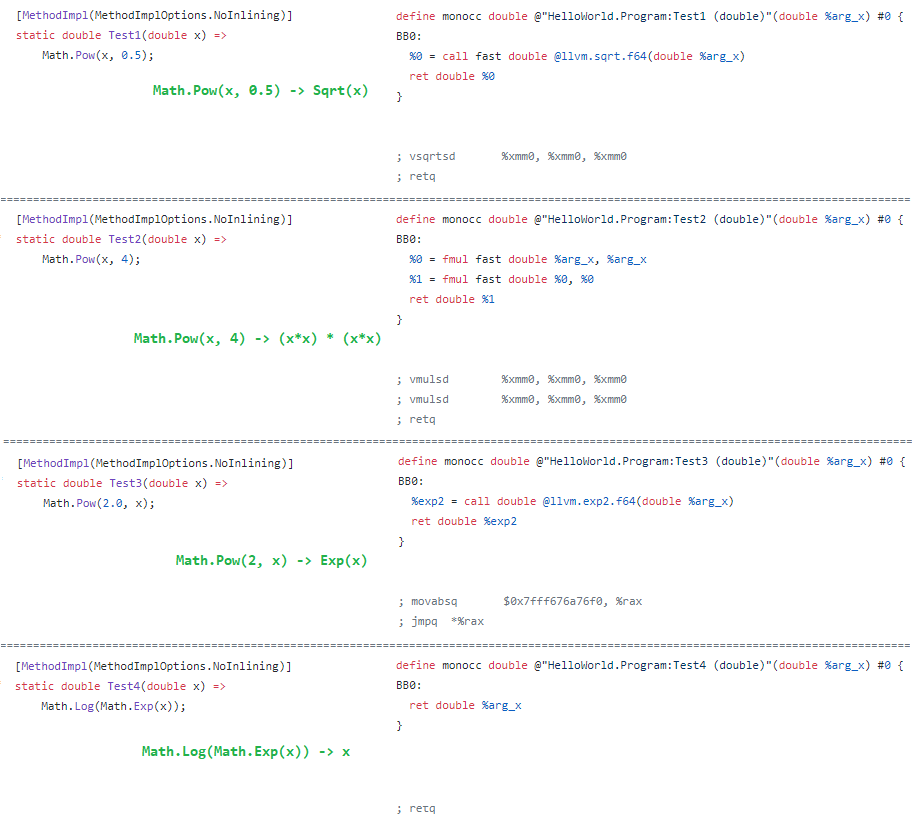{{ message }}
/ mono Public

# Implement more Math LLVM intrinsics#16578

Merged
merged 4 commits into from Sep 4, 2019
Merged

# Implement more Math LLVM intrinsics #16578

merged 4 commits into from Sep 4, 2019

## Conversation

This file contains bidirectional Unicode text that may be interpreted or compiled differently than what appears below. To review, open the file in an editor that reveals hidden Unicode characters. Learn more about bidirectional Unicode characters

###EgorBo commented Aug 29, 2019 • edited

This PR adds all missing Math/MathF intrinsics except:

1. `Round(float)` -- is not yet implemented (should not use high level `llvm.round.f32`)
2. All methods LLVM doesn't have intrinsics for, e.g. `Asin`, `Acos`, etc
3. All methods with "throw" inside, e.g. `Math.Sign`

I reorganized them a bit (grouped by parameters count and type) I suspect it's easier to look at the change like this: https://github.com/EgorBo/mono/blob/llvm-math-more2/mono/mini/intrinsics.c#L108-L245

Also, some opcodes use `OP_XXXF` for float and `OP_XXX` for double, the others use `OP_RXXX` for float and `OP_FXXX` for double so it was difficult to make it consistent (I didn't rename the existing ones).

Test:

```using System;
using System.Runtime.CompilerServices;

namespace HelloWorld
{
class Program
{
static void Main(string[] args)
{
// MathF
// (float)
Console.WriteLine(MyAbsF(-1.5f));
Console.WriteLine(MyCeilingF(1.5f));
Console.WriteLine(MyCosF(1.5f));
Console.WriteLine(MyExpF(1.5f));
Console.WriteLine(MyFloorF(1.5f));
Console.WriteLine(MyLog2F(1.5f));
Console.WriteLine(MyLog10F(1.5f));
Console.WriteLine(MySinF(1.5f));
Console.WriteLine(MySqrtF(1.5f));
Console.WriteLine(MyTruncateF(1.5f));
// (float, float)
Console.WriteLine(MyMinF(1.5f, 3));
Console.WriteLine(MyMaxF(1.5f, 3));
Console.WriteLine(MyPowF(1.5f, 3));
Console.WriteLine(MyCopySignF(1.5f, -1.5f));
// (float, float, float)

// Math
// (double)
Console.WriteLine(MyAbs(-1.5));
Console.WriteLine(MyCeiling(1.5));
Console.WriteLine(MyCos(1.5));
Console.WriteLine(MyExp(1.5));
Console.WriteLine(MyFloor(1.5));
Console.WriteLine(MyLog(1.5));
Console.WriteLine(MyLog2(1.5));
Console.WriteLine(MyLog10(1.5));
Console.WriteLine(MySin(1.5));
Console.WriteLine(MySqrt(1.5));
Console.WriteLine(MyTruncate(1.5));
// (double, double)
Console.WriteLine(MyMin(1.5, 3));
Console.WriteLine(MyMax(1.5, 3));
Console.WriteLine(MyPow(1.5, 3));
Console.WriteLine(MyCopySign(1.5, -1.5));
// (double, double, double)
}

// MathF
// (float)
[MethodImpl(MethodImplOptions.NoInlining)] static float MyAbsF(float x) => MathF.Abs(x); // should inline Math.Abs
[MethodImpl(MethodImplOptions.NoInlining)] static float MyCeilingF(float x) => MathF.Ceiling(x);
[MethodImpl(MethodImplOptions.NoInlining)] static float MyCosF(float x) => MathF.Cos(x);
[MethodImpl(MethodImplOptions.NoInlining)] static float MyExpF(float x) => MathF.Exp(x);
[MethodImpl(MethodImplOptions.NoInlining)] static float MyFloorF(float x) => MathF.Floor(x);
[MethodImpl(MethodImplOptions.NoInlining)] static float MyLog2F(float x) => MathF.Log2(x);
[MethodImpl(MethodImplOptions.NoInlining)] static float MyLog10F(float x) => MathF.Log10(x);
[MethodImpl(MethodImplOptions.NoInlining)] static float MySinF(float x) => MathF.Sin(x);
[MethodImpl(MethodImplOptions.NoInlining)] static float MySqrtF(float x) => MathF.Sqrt(x);
[MethodImpl(MethodImplOptions.NoInlining)] static float MyTruncateF(float x) => MathF.Truncate(x);
// (float, float)
[MethodImpl(MethodImplOptions.NoInlining)] static float MyMinF(float x, float y) => MathF.Min(x, y); // should inline Math.Min
[MethodImpl(MethodImplOptions.NoInlining)] static float MyMaxF(float x, float y) => MathF.Max(x, y); // should inline Math.Max
[MethodImpl(MethodImplOptions.NoInlining)] static float MyPowF(float x, float y) => MathF.Pow(x, y);
[MethodImpl(MethodImplOptions.NoInlining)] static float MyCopySignF(float x, float y) => MathF.CopySign(x, y);
// (float, float, float)
[MethodImpl(MethodImplOptions.NoInlining)] static float MyFusedMultiplyAddF(float x, float y, float z) => MathF.FusedMultiplyAdd(x, y, z);

// Math
// (double)
[MethodImpl(MethodImplOptions.NoInlining)] static double MyAbs(double x) => Math.Abs(x);
[MethodImpl(MethodImplOptions.NoInlining)] static double MyCeiling(double x) => Math.Ceiling(x);
[MethodImpl(MethodImplOptions.NoInlining)] static double MyCos(double x) => Math.Cos(x);
[MethodImpl(MethodImplOptions.NoInlining)] static double MyExp(double x) => Math.Exp(x);
[MethodImpl(MethodImplOptions.NoInlining)] static double MyFloor(double x) => Math.Floor(x);
[MethodImpl(MethodImplOptions.NoInlining)] static double MyLog(double x) => Math.Log(x);
[MethodImpl(MethodImplOptions.NoInlining)] static double MyLog2(double x) => Math.Log2(x);
[MethodImpl(MethodImplOptions.NoInlining)] static double MyLog10(double x) => Math.Log10(x);
[MethodImpl(MethodImplOptions.NoInlining)] static double MySin(double x) => Math.Sin(x);
[MethodImpl(MethodImplOptions.NoInlining)] static double MySqrt(double x) => Math.Sqrt(x);
[MethodImpl(MethodImplOptions.NoInlining)] static double MyTruncate(double x) => Math.Truncate(x);
// (double, double)
[MethodImpl(MethodImplOptions.NoInlining)] static double MyMin(double x, double y) => Math.Min(x, y);
[MethodImpl(MethodImplOptions.NoInlining)] static double MyMax(double x, double y) => Math.Max(x, y);
[MethodImpl(MethodImplOptions.NoInlining)] static double MyPow(double x, double y) => Math.Pow(x, y);
[MethodImpl(MethodImplOptions.NoInlining)] static double MyCopySign(double x, double y) => Math.CopySign(x, y);
// (double, double, double)
[MethodImpl(MethodImplOptions.NoInlining)] static double MyFusedMultiplyAdd(double x, double y, double z) => Math.FusedMultiplyAdd(x, y, z);
}
}```

Codegen:
LLVM IR (fast math): https://gist.github.com/EgorBo/219c5979272e2079b0d063ffbb7b7e19
ASM (fast math): https://gist.github.com/EgorBo/2cec0d58d00a3b19132c1d27962319c3

Some funny optimizations (with `--ffast-math`):added 4 commits Aug 29, 2019
``` Implement more Math intrinsics ```
``` 8ebf6a6 ```
``` Test on CI ```
``` 9de30eb ```
``` Update pipeline-netcore-runtime.yml ```
``` 1380861 ```
``` undo "test on CI" ```
``` f94e7cf ```
 if (in_corlib && !strcmp (m_class_get_name (cmethod->klass), "MathF") && cfg->r4fp) { // (float) if (fsig->param_count == 1 && fsig->params ->type == MONO_TYPE_R4) { if (!strcmp (cmethod->name, "Ceiling")) {

###vargaz Aug 31, 2019

It might be useful to add an intrinsics-methods.h file similar to simd-methods-netcore.h and do a binary search.

### EgorBo commented Sep 3, 2019

 @monojenkins build failed

pushed a commit to filipnavara/mono that referenced this issue Sep 4, 2019
``` Implement more Math LLVM intrinsics (mono#16578) ```
``` b4a3d9f ```
```* Implement more Math intrinsics

* Test on CI

* Update pipeline-netcore-runtime.yml

* undo "test on CI"```
pushed a commit to ManickaP/runtime that referenced this issue Jan 20, 2020
``` Implement more Math LLVM intrinsics (mono/mono#16578) ```
``` 9781613 ```
```* Implement more Math intrinsics

* Test on CI

* Update pipeline-netcore-runtime.yml

* undo "test on CI"

Commit migrated from mono/mono@30625b2```